# DAY 61 UNIT CONVERSIONS VOCABULARY Unit conversion is

• Slides: 10DAY 61 – UNIT CONVERSIONS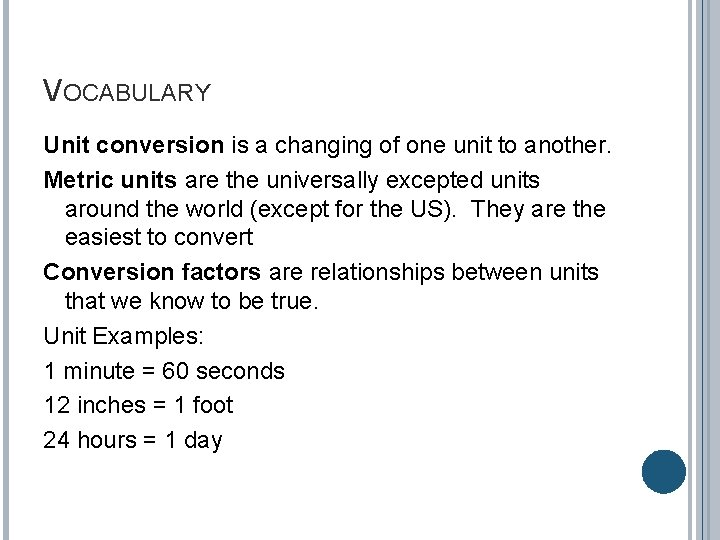VOCABULARY Unit conversion is a changing of one unit to another. Metric units are the universally excepted units around the world (except for the US). They are the easiest to convert Conversion factors are relationships between units that we know to be true. Unit Examples: 1 minute = 60 seconds 12 inches = 1 foot 24 hours = 1 daySTEPS TO CONVERTING UNITS 1. 2. 3. 4. Write the number (with units) that you want to convert. Multiply it by a conversion factor, written as a fraction. Make sure the old units cancel. Multiply all the numbers on the top and divide by the numbers on the bottom.EXAMPLE 1: CONVERT 1 M TO CM 1 m Given 100 cm 1 m For meters to cancel out, meters in the conversion factor must be on the opposite side of the fraction (fence). Conversion Factor (1 x 100) = 100 cm 1 Multiply every number on top of the fence and divide by the bottom.EXAMPLE 2: 8765 MM TO KM 8765 mm 1 cm 10 mm (8765 x 1) (10 x 100, 000) 1 km 100, 000 cm = 0. 008765 km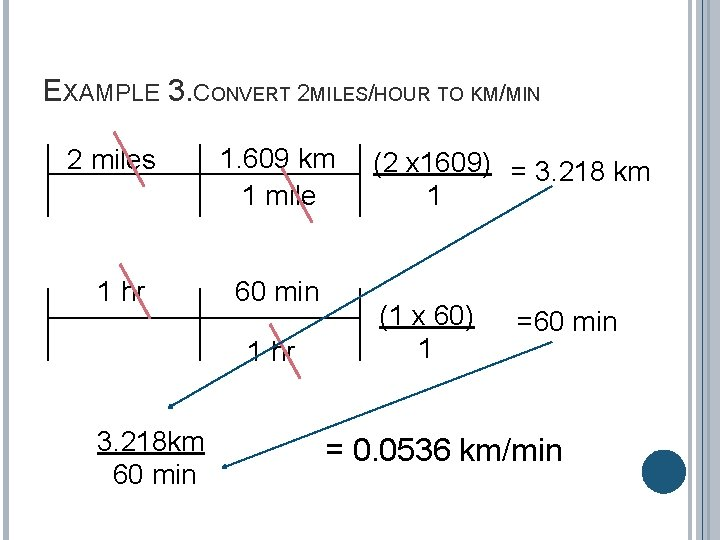EXAMPLE 3. CONVERT 2 MILES/HOUR TO KM/MIN 2 miles 1 hr 1. 609 km 1 mile 60 min 1 hr 3. 218 km 60 min (2 x 1609) = 3. 218 km 1 (1 x 60) 1 =60 min = 0. 0536 km/minTRY IT ON YOUR OWN 1. ) 12, 080 gallons per month into liters per hour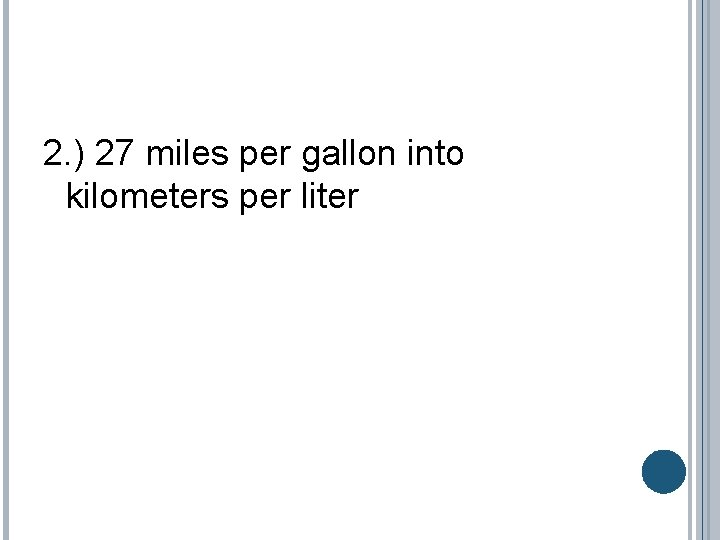2. ) 27 miles per gallon into kilometers per liter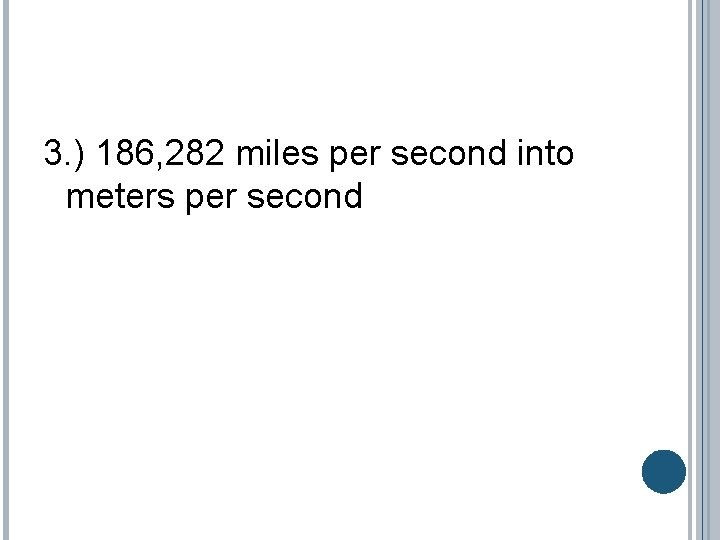3. ) 186, 282 miles per second into meters per second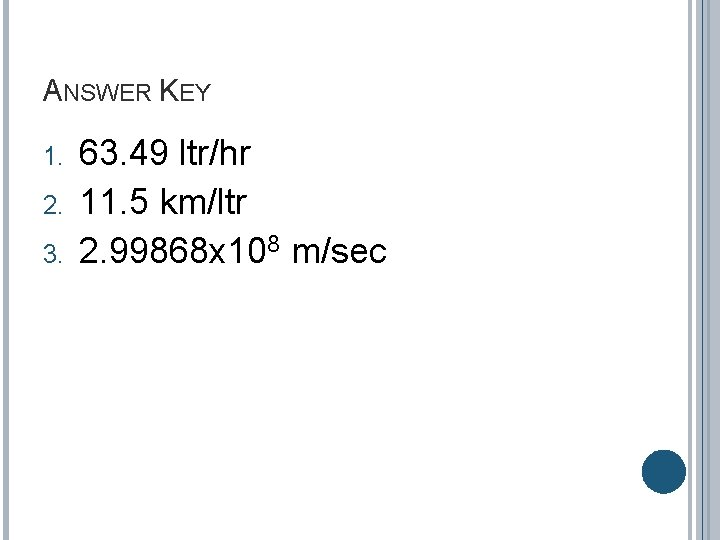ANSWER KEY 1. 2. 3. 63. 49 ltr/hr 11. 5 km/ltr 2. 99868 x 108 m/sec